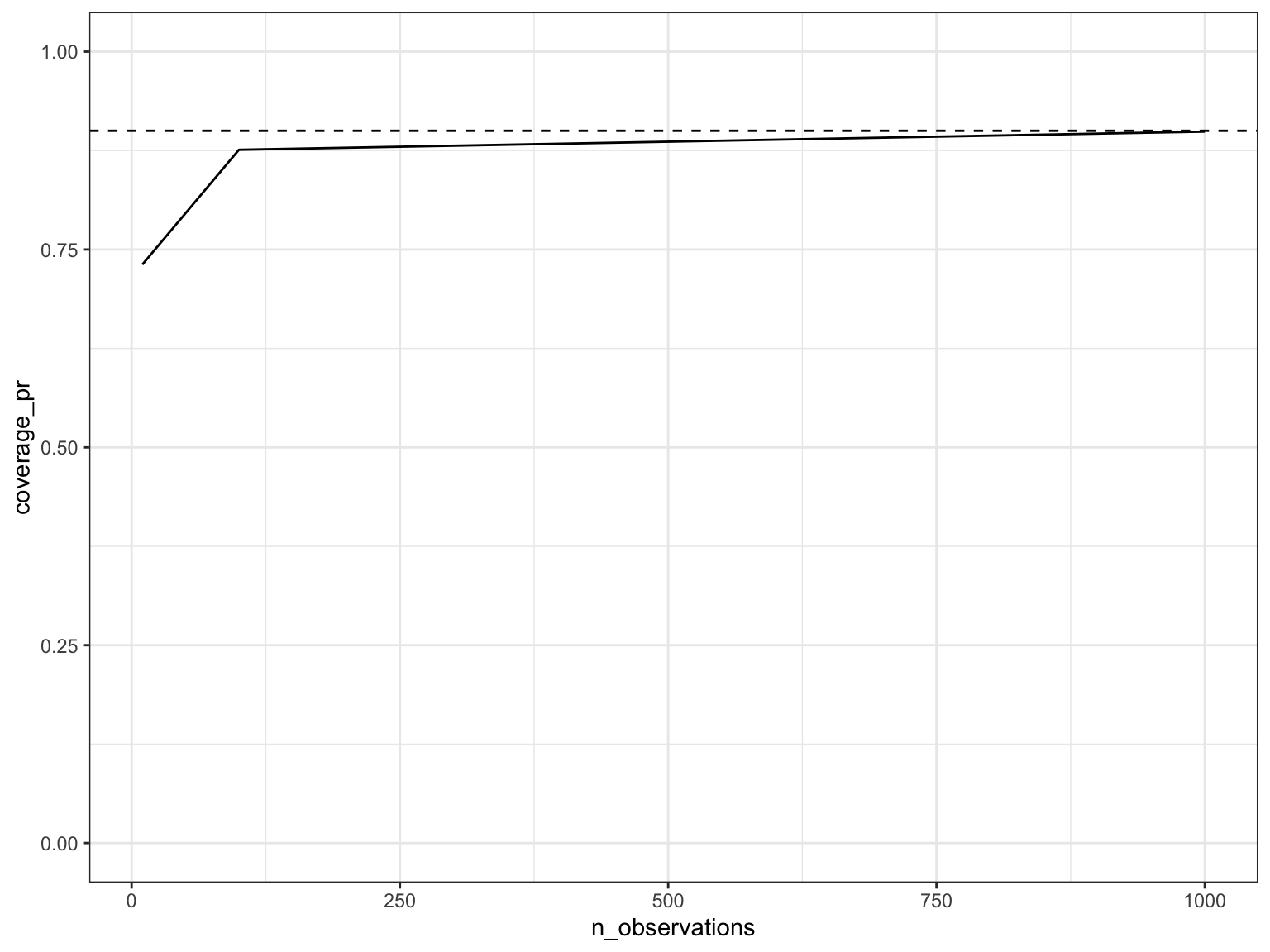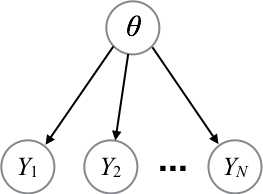# Bayesian calibration, continued

• Setup: credible intervals in a simple beta binomial model
• Use the well-specified setup described earlier
• first, with a large dataset ($$n = 10000$$)
• second, with a small dataset ($$n = 2$$)
• Use simulations to speculate on the calibration of the credible interval in the two setups ($$n = 10000$$ and $$n=2$$)

Exercise debrief

• Suprise!!: in a Bayesian well-specified context, calibration holds for all dataset sizes!
• Contrast with typical frequentist intervals, where approximate calibration is only achieved for large enough dataset size
• This is because frequentist intervals need to rely on asymptotics to be computable (Central Limit Theorem)
• Next:
• why well specified Bayesian models are calibrated
• two applications of this calibration property

# Why well-specified credible intervals are calibrated for any fixed dataset sizes• Denote the data by $$X$$
• Parameter of interest: $$I$$
• Denote the credible interval by $$C(X)$$
• We want to show $${\mathbb{P}}^*(I \in C(X)) = 0.9$$
• By construction, the interval satisfies, for all observed data $$x$$, $${\mathbb{P}}(I \in C(x) | X = x) = 0.9$$
• Recall the law of total probability:
• for any partition $$E_i$$ of the sample space,
• we have $${\mathbb{P}}(A) = \sum_i {\mathbb{P}}(A, E_i)$$
• Here: $$A = (I \in C(X))$$, $$E_i = (X = x_i)$$
• where $$\{x_i\}$$ denotes the possible values the data can potentially take across all possible simulated experiments.
• Putting it all together and applying chain rule: \begin{align*} {\mathbb{P}}^*(I \in C(X)) &= \sum_i {\mathbb{P}}^*(I \in C(X), X = x_i) \\ &= \sum_i {\mathbb{P}}^*(X = x_i) {\mathbb{P}}^*(I \in C(x_i) | X = x_i) \\ &= \sum_i {\mathbb{P}}^*(X = x_i) {\mathbb{P}}(I \in C(x_i) | X = x_i) \quad\quad \text{(assuming model is well-specified)} \\ &= \sum_i {\mathbb{P}}^*(X = x_i) 0.9 \\ &= 0.9 \sum_i {\mathbb{P}}^*(X = x_i) = 0.9. \end{align*}

# Application 1: software testing

• Using calibration is a great way to test correctness of your software implementation.
• Correct Bayesian inference code on correctly generated data (based on same model): calibrated.
• Buggy Bayesian inference code: almost never calibrated.
• It is nice because you can run your test on small dataset where inference is fast.
• Pick the test just big enough to get code coverage.
• There is a way to make this idea even more powerful in the context of MCMC (see reading “Getting it right” and Cook et al.)

• Note:
• People often use “synthetic data” as follows:
• Generate data, hold out the true parameter $$\theta^\star$$
• Report distance between inferred $$\theta$$ and $$\theta^\star$$
• Now how to interpret this distance? Could be unclear.
• Message here: there are better ways to use synthetic data in a Bayesian context!

# Application 2: goodness-of-fit

• Goodness of fit: is the model any good?
• Related to the question of model selection, but distinct
• In contrast to model selection which involves two or more models, goodness-of-fit involves only one model
• Note: in practice, we know the vast majority of the models are technically misspecified, so what is the point? (obligatory George Box quote: “All models are wrong, but some are useful”)
• The procedure will give also an intuitive quantification of the degree of misspecification
• With experience, you will get some sense of the point where models are “well-specified enough to be useful”
• Setup:
• assume simple setup:
• we have many replicates of a dataset $$y^{(1)}, \dots, y^{(100)}$$ (use $$d$$ to index dataset)
• each one has say $$n=20$$ observations in it $$y^{(d)} = (y^{(d)}_1, y^{(d)}_2, \dots, y^{(d)}_{20})$$
• let $$C$$ denote a 90% credible interval for $$Y_1$$

### Poll: how to use calibration to do goodness-of-fit

1. Use synthetic data, loop over $$i$$: check if $$\frac{1}{100} \sum_d {{\bf 1}}[y^{(d)}_1 \in C(y^{(d)}_1, \dots, y^{(d)}_{20})] \approx 0.9$$
2. Use synthetic data, loop over $$i$$: check if $$\frac{1}{100} \sum_d {{\bf 1}}[y^{(d)}_1 \in C(y^{(d)}_2, \dots, y^{(d)}_{20})] \approx 0.9$$
3. Use real data, loop over $$i$$: check if $$\frac{1}{100} \sum_d {{\bf 1}}[y^{(d)}_1 \in C(y^{(d)}_1, \dots, y^{(d)}_{20})] \approx 0.9$$
4. Use real data, loop over $$i$$: check if $$\frac{1}{100} \sum_d {{\bf 1}}[y^{(d)}_1 \in C(y^{(d)}_2, \dots, y^{(d)}_{20})] \approx 0.9$$
5. None of the above

Recall: $${{\bf 1}}[\cdots]$$ denotes the indicator on the event $$[\cdot]$$, i.e. a function that is 1 if the input outcome $$s$$ is in the event, 0 otherwise.

• Main idea:
• define $$y_{-i} = (y_1, y_2, y_{i-1}, y_{i+1}, \dots, y_{20})$$ (note: related ideas are used in machine learning to assess generalization capability, this is related but distinct; here we want a yes-no answer to “is the model well-specified?”)
• if the model is well-specified, $${\mathbb{P}}(Y_{i} \in C_\alpha(Y_{-i})) = 0.9$$
• No assumptions on the structure of the model.
• Works for any model! Time series, spatial, etc.
• Encourage your applied collaborators to do replicates!
• In the last question, we approximated the probability above by averaging over datasets

• If you don’t have many datasets, you can average over $$i$$ (this is called “leave one out” LOO) but this is more subtle as you loose iid (so the properties of the goodness of fit procedure will be more dependent on the model), this leads to ${\mathbb{P}}(Y_i \in C_\alpha(Y_{-i})) \approx \frac{1}{n} \sum_i {{\bf 1}}[y_{i} \in C_\alpha(y_{-i})]$

• Computational challenge: leave one out requires computation of $$n$$ posterior distributions, that seems hopelessly expensive
• Insight: all these posterior distributions are very similar
• Classical method that use this: importance sampling. See for example https://link.springer.com/article/10.1007/s11222-016-9696-4
• Project idea: leverage modern methods to compute challenging leave one out problems, e.g. using sequential change of measure or non-reversible parallel tempering (which will be covered in the second half of the course)
• Picking a $$\alpha = 0.9$$ credible interval is arbitrary
• Instead: do the same as before but for all $$\alpha \in [0, 1]$$,
• let $$C_\alpha$$ denote an $$\alpha$$ credible interval
• get fraction $$f(\alpha)$$ for each $$\alpha$$: $f(\alpha) = \frac{1}{100} \sum_d {{\bf 1}}[y^{(d)}_1 \in C_\alpha(y^{(d)}_2, \dots, y^{(d)}_{20})]$

### Poll: if the model is well-specified, what do you expect to see

1. $$f(\alpha)$$ should be constant
2. $$f(\alpha) = \alpha$$
3. $$f(\alpha) = a \alpha$$ for some $$a$$
4. $$f(\alpha) = a \alpha + b$$ for some $$a, b$$
5. Something else
• Note that the function $$f(\alpha) = \alpha$$ is the CDF of the uniform $$[0, 1]$$ distribution
• In the special case where $$C_\alpha = F^{-1}_{y_1|y_2, \dots, y_n}(\alpha)$$, this is closely related to the probability integral transform (PIT)
• If $$F$$ is the CDF of a random variable $$X$$, then $$F(X)$$ is uniform (if $$F$$ monotone strictly increasing, this is simple to see: $${\mathbb{P}}(F(X) \le \alpha) = {\mathbb{P}}(X \le F^{-1}(\alpha)) = F(F^{-1}(\alpha)) = \alpha$$, but this is always true)
• Here, $$X$$ is distributed according to the posterior distribution, and $$F$$ is the CDF of the posterior, used to construct the credible interval
• PIT is related to the more commonly used but less formal “posterior predictive check”, where data point $$i$$ is not excluded from the conditioning (e.g. answer 3 in the first poll, or leave one out version)
• easier to compute (only one posterior instead of $$n$$ posteriors)
• no theoretical guarantees and hence harder to interpret
• useful as a quick and dirty visualization trick

# Misspecified models and calibration

What to do if your model is misspecified?

One obvious possibility is to make the model better. But in the following we show that under certain conditions, another possibility is to collect more data.

• Now suppose we are using the “wrong prior”, i.e. data generation uses uniform prior but we base or posterior computation on the non-uniform prior from earlier

• Again, let’s do it for small (10 launches), medium (100), and large datasets (1000), plotting the nominal coverage (dashed) against the actual coverage (solid line)

 # Using now the same non-uniform prior as before for posterior calculation
K <- 1000

rdunif <- function(max) { return(ceiling(max*runif(1))) }

posterior_distribution <- function(prior_probs, n_successes, n_trials){
K <- length(prior_probs)-1 # K+1 values that your p can assume
n_fails <- n_trials - n_successes
p <- seq(0, 1, 1/K)
posterior_probs <-                                       # 1. this computes gamma(i)
prior_probs *                                          #    - prior
p^n_successes * (1-p)^n_fails                          #    - likelihood
posterior_probs <- posterior_probs/sum(posterior_probs)  # 2. normalize gamma(i)
post_prob <- rbind(p, posterior_probs)
return(post_prob)
}

high_density_intervals <- function(alpha, posterior_probs){
ordered_probs = posterior_probs[,order(posterior_probs[2,], decreasing = TRUE)]
cumulative_probs = cumsum(ordered_probs[2,])
index = which.max(cumulative_probs >= (1-alpha))
return(ordered_probs[,1:index, drop=FALSE])
}

hdi_coverage_pr <- function(n_datapoints) {
n_inclusions <- 0
for (repetition in seq(1:n_repeats)) {
i <- rdunif(K + 1) - 1  # Always generate the data using a uniform prior
true_p <- i/K
x <- rbinom(1, n_datapoints, true_p)
post <- posterior_distribution(prior_used_for_computing_posterior, x, n_datapoints)

# This if is just a hacky way to check if true parameter is in the HDI credible interval
if (sum(abs(true_p - high_density_intervals(alpha, post)[1,]) < 10e-10) == 1) {
n_inclusions <- n_inclusions + 1
}
}
return(n_inclusions/n_repeats) # Fraction of simulation where the true parameter was in interval
}

prior_used_for_computing_posterior <- dnorm(seq(0, 1, 1/K),mean = 0.2, sd=0.2)
prior_used_for_computing_posterior <- prior_used_for_computing_posterior / sum(prior_used_for_computing_posterior)

set.seed(1)

n_repeats <- 1000
alpha <- 0.1

df <- data.frame("n_observations" = c(10, 100, 1000))
df$coverage_pr <- sapply(df$n_observations, hdi_coverage_pr)

ggplot(data=df, aes(x=n_observations, y=coverage_pr)) +
ylim(0, 1) +
geom_hline(yintercept=1-alpha, linetype="dashed", color = "black") +
geom_line()• Bad news: for small datasets we are no longer calibrated, in the worst possible way
• Higher than dash line: would have meant inference is being conservative, i.e. more right than it actually claimed. That’s not too bad.
• Lower than dash line: we are being overconfident or anti-conservative, which in some case can be reckless
• Good news: this gets quickly corrected as dataset gets larger. Why?

# Asymptotic calibration under certain misspecification

• There is no general calibration theory for misspecified models, only special cases (outside of that, use simulation studies or cross-validation techniques)
• Setup in which we have a theorem: graphical model of the following formBernstein-von Mises: under certain conditions, even when the prior is misspecified the actual coverage of credible intervals converges to the nominal coverage. (converge just means “gets arbitrarily close”)

Conditions include, but are not limited to:

1. $$\theta \in \Theta$$ needs to live in a continuous rather than discrete space! I.e.: $$\Theta \subset {\mathbf{R}}^d$$
• Intuition: Bernstein-von Mises actually proves something stronger: convergence of the rescaled, centered posterior to a normal distribution, which is a continuous distribution (can you guess the scaling?)
• “Bayesian Central Limit Theorem”
2. $$p(\theta)$$ (the prior) is now a density instead of a PMF,
• we assume it is non-zero in a neighborhood of the true parameter
3. we assume the posterior mean is consistent
• recall: that means the error goes to zero as number of data points increases
4. we assume the model is parametric:
• $$\theta$$ is allowed to be a vector, but you are not allowed to make it bigger as the number of observations increases

Practical consequence:

• for large datasets, and under certain condition, the prior is “washed away” by the likelihood
• The conditions are not crazy and hold in some situations of interest
• But there are also simple practical situations where the conditions do not hold (e.g. prior assigning zero mass to important regions)